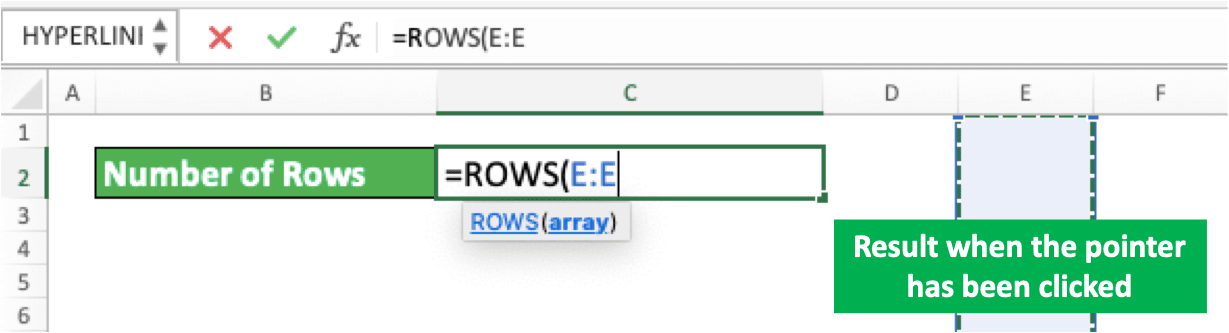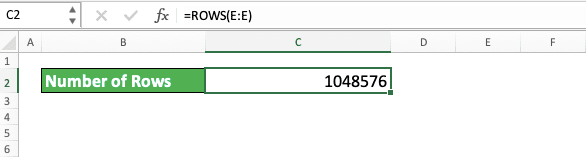Number of Columns and Rows in Excel - Compute Expert

# Number of Columns and Rows in Excel

Home >> Excel Tutorials from Compute Expert >> Excel Tips and Trick >> Number of Columns and Rows in Excel

In this article, you will know the number of rows and columns provided by excel in its worksheet. Besides that, we will explain how to use that number in your cell/formula. We will also discuss how to get to the edge of your row/column easily.

Sometimes, it is important to know how many excel rows and columns we have to plan our input and data processing. Each excel version has its own number of rows and columns. If you need to know the number for some purposes of yours, then you have come to the right place!

Disclaimer: This post may contain affiliate links from which we earn commission from qualifying purchases/actions at no additional cost for you. Learn more

## The Number of Columns and Rows in Excel 2007, 2010, 2013, 2016, and Others

Here is a table that gives complete information on the maximum number of columns and rows in various Excel versions.

Excel VersionNumber of ColumnsNumber of Rows
Excel 525616,384
Excel 9525616,384
Excel 9725665,356
Excel 200025665,356
Excel 2002/XP25665,356
Excel 200325665,356
Excel 200716,3841,048,576
Excel 201016,3841,048,576
Excel 201316,3841,048,576
Excel 201616,3841,048,576
Excel 201916,3841,048,576

As you can see in the table, excel doesn’t change its worksheet’s number of rows and columns since excel 2007. However, I think the rows/columns are already enough to be used in our data input and processing process!

## How to Get the Number of Rows and Columns in Your Worksheet

Want to get the number that represents the maximum number of rows and columns in your worksheet? Maybe you want to use it as a cell content or as a reference for a formula. This can be done easily if you know the method.

Then, what is the method? The method is by using ROWS and COLUMNS formulas available in excel. ROWS can help us count the number of rows in a cell range while COLUMNS count the columns.

To get the number of rows in an excel worksheet using ROWS, we need to input a whole column’s cell range. We can input it by clicking on the excel column letter at the top or by typing it manually. Input whichever column in the excel worksheet to get the number of rows you need.

For the column cell range input by clicking on the column letter, here are the illustrations in screenshots.To type it manually, here is the illustration. You must type a letter, a colon (:), and the letter again in your ROWS.Both input ways in ROWS will produce the same result as you can see below.You have got your maximum number of rows in a worksheet!

Then, what if what you want is the number of columns? Use COLUMNS to get the number.

The use of COLUMNS is similar to ROWS. However, here, you need to input the cell range of a whole row in your worksheet in excel. The way is by clicking the excel row number on the left or by typing the coordinate manually.

Here are the screenshots to illustrate how to input row cell range by clicking on the row number.And, here is the manual typing input method. Similar to getting the number of rows, you must type a number, colon, and the number again in your COLUMNS.Both COLUMNS input methods will give the same result.The number produced depends on the worksheet’s maximum number of columns in your excel.

## How to Go to the Edge of Your Worksheet’s Row and/or Column

What if you want to go to the edge of your excel worksheet’s row and/or column? It is easy! You just need to use special shortcut buttons from excel to do it.

To reach the column edge, place a cell cursor where there is no data on your right in the same row. Then, press Ctrl + right directional buttons (Command + right directional buttons on Mac)

The result will be like this.If you want to reach the row edge, place a cursor where there is no data below in the same column. Then, press Ctrl + down directional buttons (Command + down directional buttons on Mac)

The result will be like this.Your cell cursor will automatically move to the edge of your worksheet!

If you do Insert Row or Insert Column, it won’t add the number of rows or columns in your worksheet! Doing that will only make the blank rows or columns on the edge move to where you insert rows or columns.

The same principle also happens when you do Delete Row or Delete Column.

Related tutorials you should learn too:

Get updated excel info from Compute Expert by registering your email. It's free!